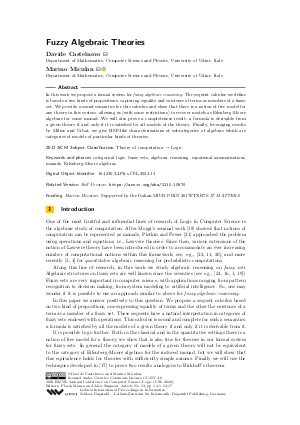Document# Fuzzy Algebraic Theories

### Authors Davide Castelnovo, Marino Miculan## File

LIPIcs.CSL.2022.13.pdf
• Filesize: 0.88 MB
• 17 pages

## Cite As

Davide Castelnovo and Marino Miculan. Fuzzy Algebraic Theories. In 30th EACSL Annual Conference on Computer Science Logic (CSL 2022). Leibniz International Proceedings in Informatics (LIPIcs), Volume 216, pp. 13:1-13:17, Schloss Dagstuhl - Leibniz-Zentrum für Informatik (2022)
https://doi.org/10.4230/LIPIcs.CSL.2022.13

## Abstract

In this work we propose a formal system for fuzzy algebraic reasoning. The sequent calculus we define is based on two kinds of propositions, capturing equality and existence of terms as members of a fuzzy set. We provide a sound semantics for this calculus and show that there is a notion of free model for any theory in this system, allowing us (with some restrictions) to recover models as Eilenberg-Moore algebras for some monad. We will also prove a completeness result: a formula is derivable from a given theory if and only if it is satisfied by all models of the theory. Finally, leveraging results by Milius and Urbat, we give HSP-like characterizations of subcategories of algebras which are categories of models of particular kinds of theories.

## Subject Classification

##### ACM Subject Classification
• Theory of computation → Logic
##### Keywords
• categorical logic
• fuzzy sets
• algebraic reasoning
• equational axiomatisations
• Eilenberg-Moore algebras

## Metrics

• Access Statistics
• Total Accesses (updated on a weekly basis)
0

## References

1. N. Ajmal. Homomorphism of fuzzy groups, correspondence theorem and fuzzy quotient groups. Fuzzy sets and systems, 61(3):329-339, 1994.2. N. Ajmal and A. S. Prajapati. Fuzzy cosets and fuzzy normal subgroups. Information sciences, 64(1-2):17-25, 1992.3. G. Bacci, R. Mardare, P. Panangaden, and G. Plotkin. An algebraic theory of Markov processes. In 33rd Symposium on Logic in Computer Science (LICS), pages 679-688, 2018.4. G. Bacci, R. Mardare, P. Panangaden, and G. Plotkin. Quantitative equational reasoning. Foundations of Probabilistic Programming, page 333, 2020.5. G. Birkhoff. On the structure of abstract algebras. Proceedings of the Cambridge Philosophical Society, 10:433-454, 1935.6. Filippo Bonchi, Barbara König, and Daniela Petrisan. Up-to techniques for behavioural metrics via fibrations. In CONCUR, volume 118 of LIPIcs, pages 17:1-17:17. Schloss Dagstuhl - Leibniz-Zentrum für Informatik, 2018.7. F. Borceux. Handbook of Categorical Algebra: Volume 2, Categories and Structures, volume 2. Cambridge University Press, 1994.8. Davide Castelnovo and Marino Miculan. Fuzzy algebraic theories. CoRR, abs/2110.10970, 2021. URL: http://arxiv.org/abs/2110.10970.
9. M. P Fourman and D. S. Scott. Sheaves and logic. In Applications of sheaves, pages 302-401. Springer, 1979.10. Martin Hyland and John Power. Discrete lawvere theories and computational effects. Theoretical Computer Science, 366(1-2):144-162, 2006.11. Martin Hyland and John Power. The category theoretic understanding of universal algebra: Lawvere theories and monads. Electron. Notes Theor. Comput. Sci., 172:437-458, 2007. URL: https://doi.org/10.1016/j.entcs.2007.02.019.
12. Peter T Johnstone. Stone spaces, volume 3. Cambridge University Press, 1982.13. Gregory Maxwell Kelly. A note on relations relative to a factorization system. In Category Theory, pages 249-261. Springer, 1991.14. S. MacLane. Categories for the working mathematician, volume 5. Springer Science & Business Media, 2013.15. R. Mardare, P. Panangaden, and G. Plotkin. On the axiomatizability of quantitative algebras. In 32nd Symposium on Logic in Computer Science (LICS), pages 1-12. IEEE, 2017.16. A. S. Mashour, M. H. Ghanim, and F. I. Sidky. Normal fuzzy subgroups. Information Sciences, 20:53-59, 1990.17. S. Milius and H. Urbat. Equational axiomatization of algebras with structure. In International Conference on Foundations of Software Science and Computation Structures, pages 400-417. Springer, 2019.18. Eugenio Moggi. Notions of computation and monads. Inf. Comput., 93(1):55-92, 1991. URL: https://doi.org/10.1016/0890-5401(91)90052-4.
19. J. N. Mordeson, D. S. Malik, and N. Kuroki. Fuzzy semigroups, volume 131. Springer, 2012.20. K. Nishizawa and J. Power. Lawvere theories enriched over a general base. Journal of Pure and Applied Algebra, 213(3):377-386, 2009.21. Gordon D. Plotkin and John Power. Notions of computation determine monads. In FoSSaCS, volume 2303 of Lecture Notes in Computer Science, pages 342-356. Springer, 2002.22. Gordon D. Plotkin and John Power. Algebraic operations and generic effects. Appl. Categorical Struct., 11(1):69-94, 2003. URL: https://doi.org/10.1023/A:1023064908962.
23. John Power. Discrete lawvere theories. In International Conference on Algebra and Coalgebra in Computer Science, pages 348-363. Springer, 2005.24. A. Rosenfeld. Fuzzy groups. Journal of mathematical analysis and applications, 35(3):512-517, 1971.25. N. H. Williams. On Grothendieck universes. Compositio Mathematica, 21(1):1-3, 1969.26. O. Wyler. Lecture notes on topoi and quasitopoi. World Scientific, 1991.27. O. Wyler. Fuzzy logic and categories of fuzzy sets. In Non-Classical Logics and Their Applications to Fuzzy Subsets, pages 235-268. Springer, 1995.X

Feedback for Dagstuhl Publishing